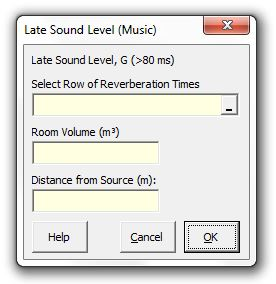### Strutt Help

Late Sound Level (Music)    1/1, 1/3

Strutt|Auditorium Acoustics|Late Sound Level (Music) calculates the late G_(80ms-oo) component of the sound field in an auditorium using Barron's revised theory.Revised theory accounts for the decrease in the strength of the reflected sound with distance which occurs with distance in large(r) auditoria - i.e. the reverberant sound component is not constant when using revised theory, but decays exponentially with distance from the source.

The calculation assumes an omnidirectional point source and diffuse conditions in the auditorium.

Note that the resulting level is expressed as strength (G) and can be converted to 'relative loudness' (i.e. relative to the source L_W) by subtracting 31 dB.

The late reflections (after 80 ms) are calculated using:

l=31200/V e^(-(0.04 r)/T) e^(-1.11/T), where

r is the distance from source.
V is the volume of the auditorium.
T is the reverberation time.

Strutt calculates the late sound level as:

G_(80ms-oo)=10log(l)

Reference: Barron Auditorium Acoustics and Architectural Design, 2nd edition, Appendix B.3